Скачать презентацию Conic Sections Mathworld Circle Circle

1f82e8f21d2b1eb68c9fe03cbb0d3542.ppt

• Количество слайдов: 44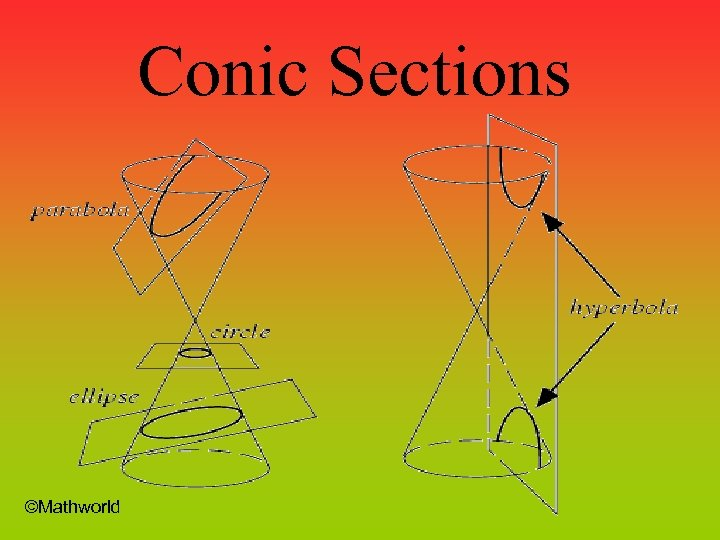Conic Sections ©Mathworld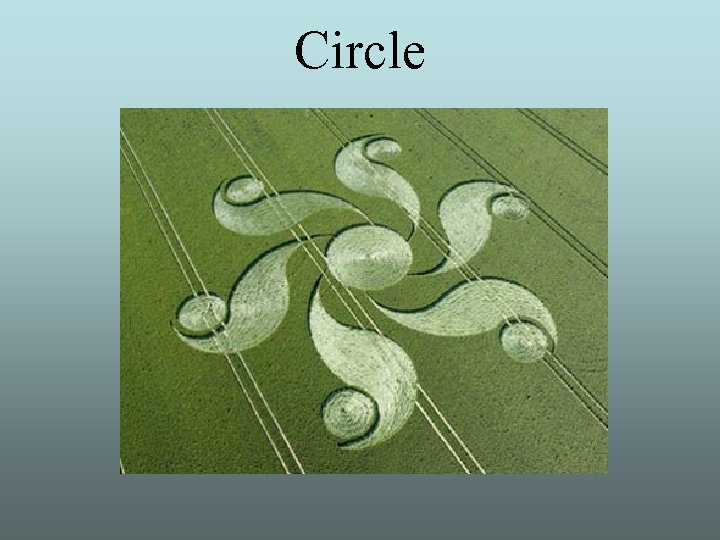Circle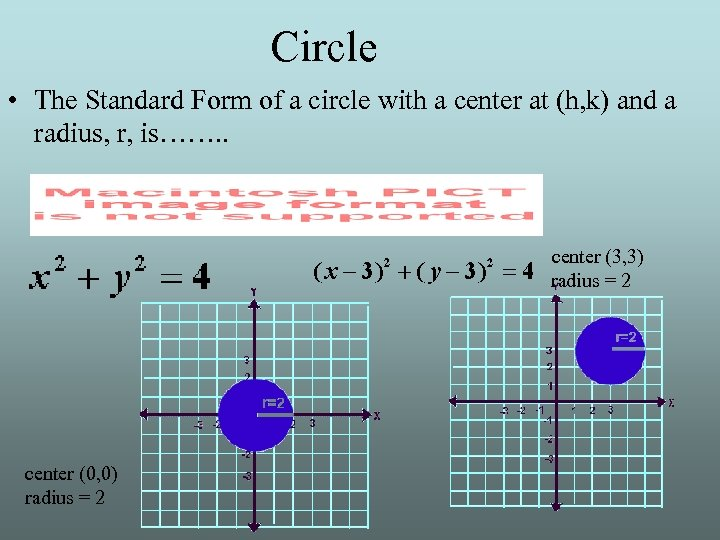Circle • The Standard Form of a circle with a center at (h, k) and a radius, r, is……. . center (3, 3) center (0, 0) radius = 2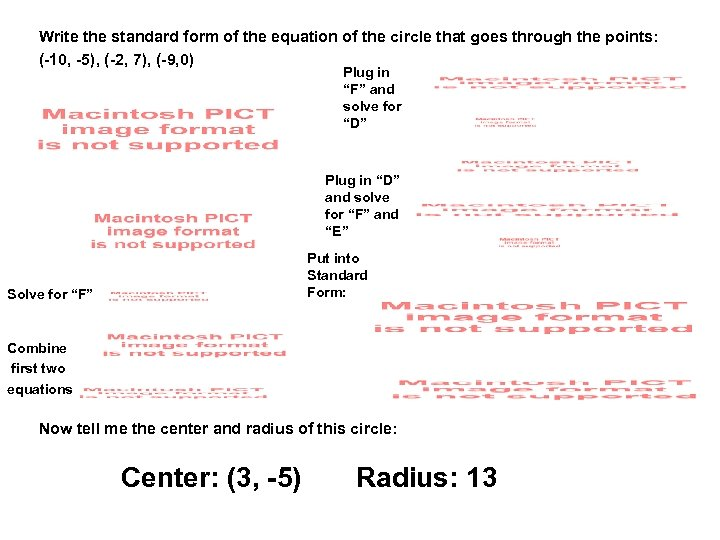Write the standard form of the equation of the circle that goes through the points: (-10, -5), (-2, 7), (-9, 0) Plug in “F” and solve for “D” Plug in “D” and solve for “F” and “E” Put into Standard Form: Solve for “F” Combine first two equations Now tell me the center and radius of this circle: Center: (3, -5) Radius: 13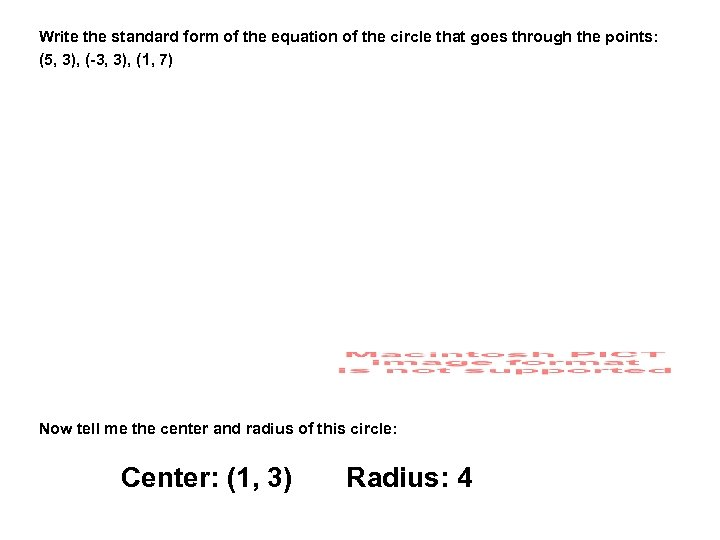Write the standard form of the equation of the circle that goes through the points: (5, 3), (-3, 3), (1, 7) Now tell me the center and radius of this circle: Center: (1, 3) Radius: 4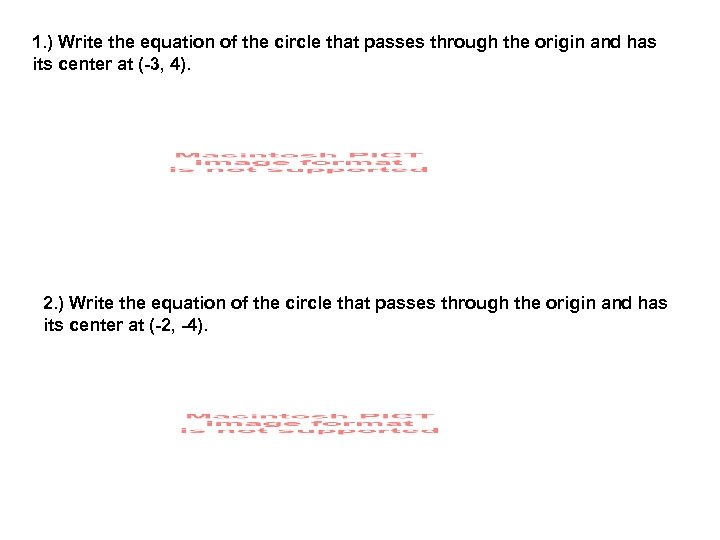1. ) Write the equation of the circle that passes through the origin and has its center at (-3, 4). 2. ) Write the equation of the circle that passes through the origin and has its center at (-2, -4).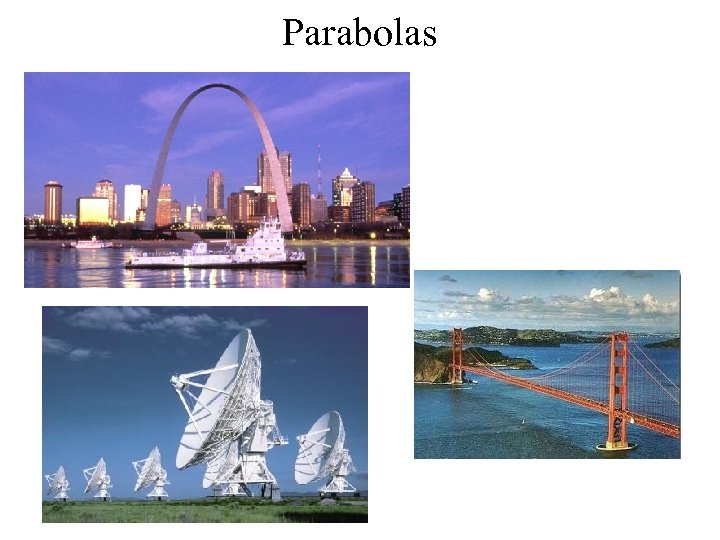Parabolas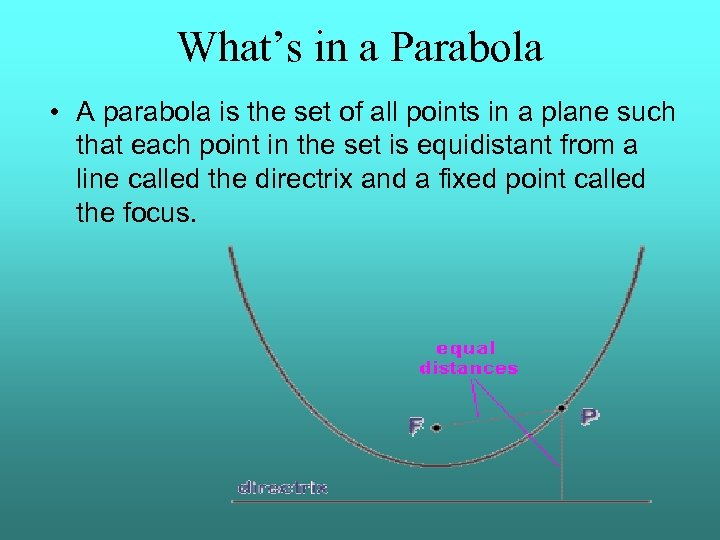What’s in a Parabola • A parabola is the set of all points in a plane such that each point in the set is equidistant from a line called the directrix and a fixed point called the focus.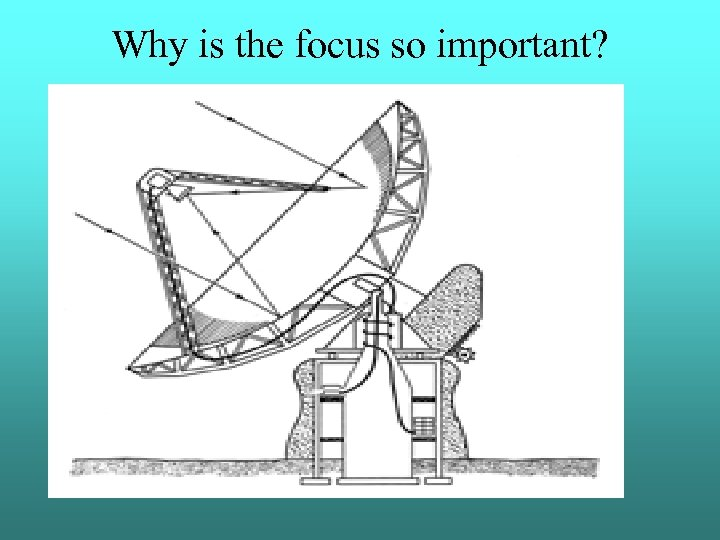Why is the focus so important?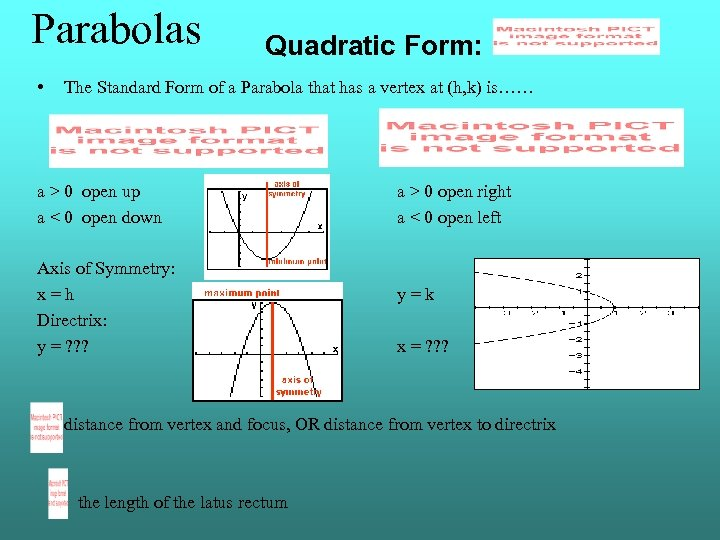Parabolas • Quadratic Form: The Standard Form of a Parabola that has a vertex at (h, k) is…… a > 0 open up a < 0 open down Axis of Symmetry: x = h Directrix: y = ? ? ? a > 0 open right a < 0 open left y = k x = ? ? ? distance from vertex and focus, OR distance from vertex to directrix the length of the latus rectum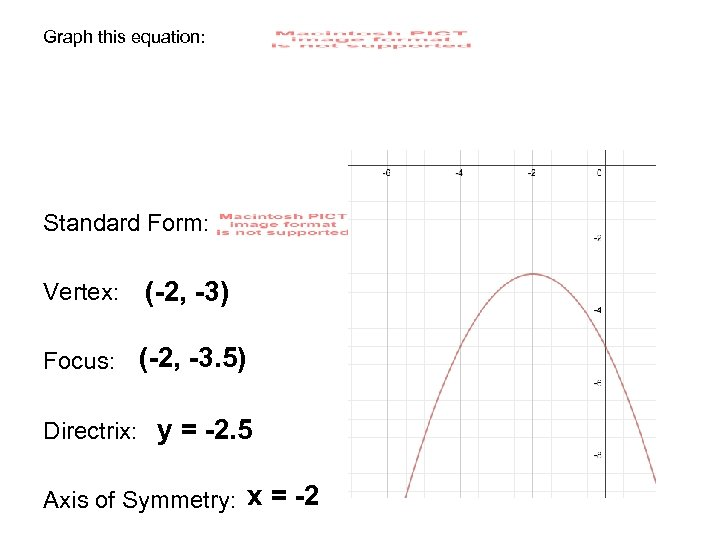Graph this equation: Standard Form: Vertex: (-2, -3) Focus: (-2, -3. 5) Directrix: y = -2. 5 Axis of Symmetry: x = -2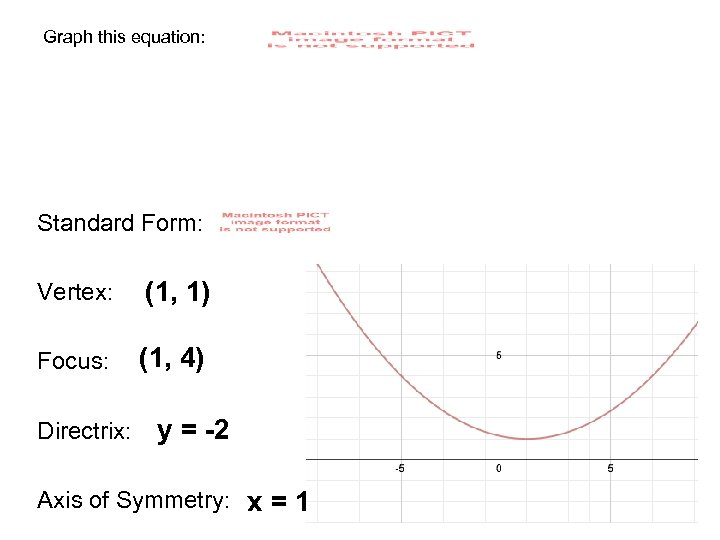Graph this equation: Standard Form: Vertex: (1, 1) Focus: (1, 4) Directrix: y = -2 Axis of Symmetry: x = 1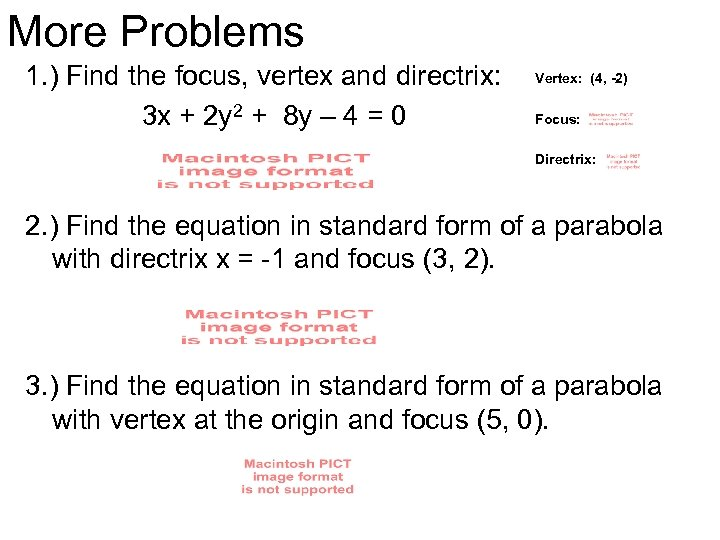More Problems 1. ) Find the focus, vertex and directrix: 3 x + 2 y 2 + 8 y – 4 = 0 Vertex: (4, -2) Focus: Directrix: 2. ) Find the equation in standard form of a parabola with directrix x = -1 and focus (3, 2). 3. ) Find the equation in standard form of a parabola with vertex at the origin and focus (5, 0).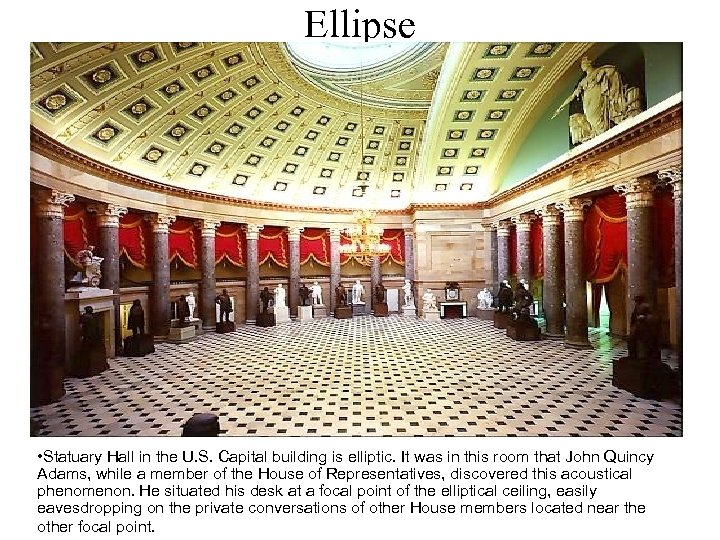Ellipse • Statuary Hall in the U. S. Capital building is elliptic. It was in this room that John Quincy Adams, while a member of the House of Representatives, discovered this acoustical phenomenon. He situated his desk at a focal point of the elliptical ceiling, easily eavesdropping on the private conversations of other House members located near the other focal point.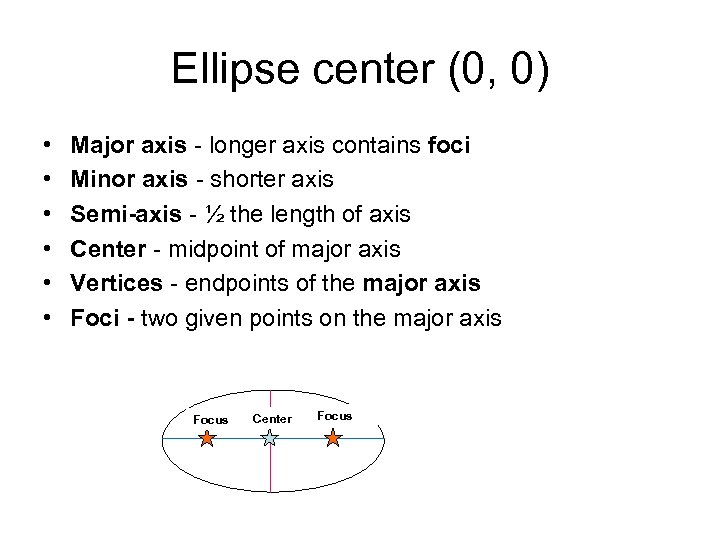Ellipse center (0, 0) • • • Major axis - longer axis contains foci Minor axis - shorter axis Semi-axis - ½ the length of axis Center - midpoint of major axis Vertices - endpoints of the major axis Foci - two given points on the major axis Focus Center Focus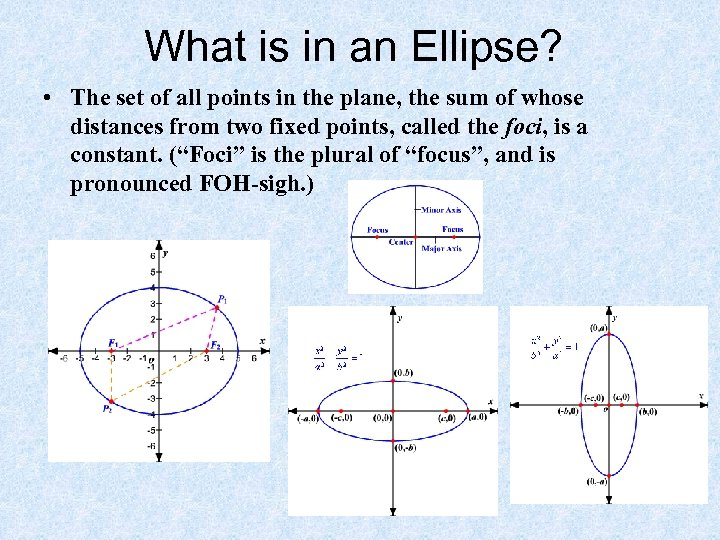What is in an Ellipse? • The set of all points in the plane, the sum of whose distances from two fixed points, called the foci, is a constant. (“Foci” is the plural of “focus”, and is pronounced FOH-sigh. )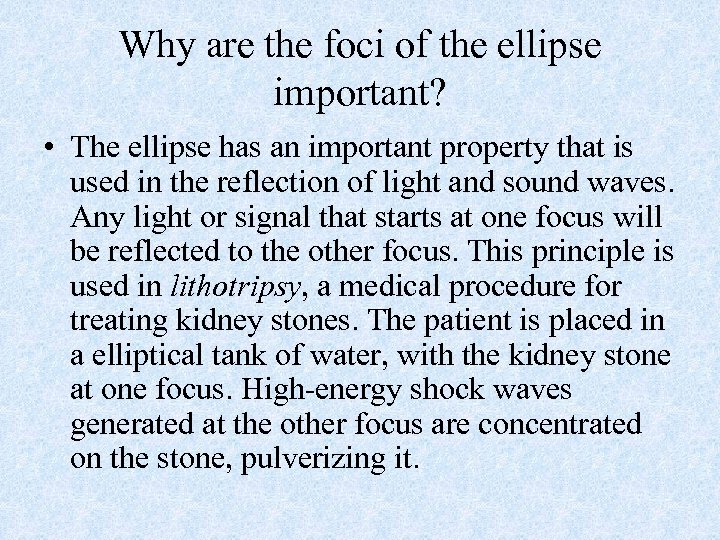Why are the foci of the ellipse important? • The ellipse has an important property that is used in the reflection of light and sound waves. Any light or signal that starts at one focus will be reflected to the other focus. This principle is used in lithotripsy, a medical procedure for treating kidney stones. The patient is placed in a elliptical tank of water, with the kidney stone at one focus. High-energy shock waves generated at the other focus are concentrated on the stone, pulverizing it.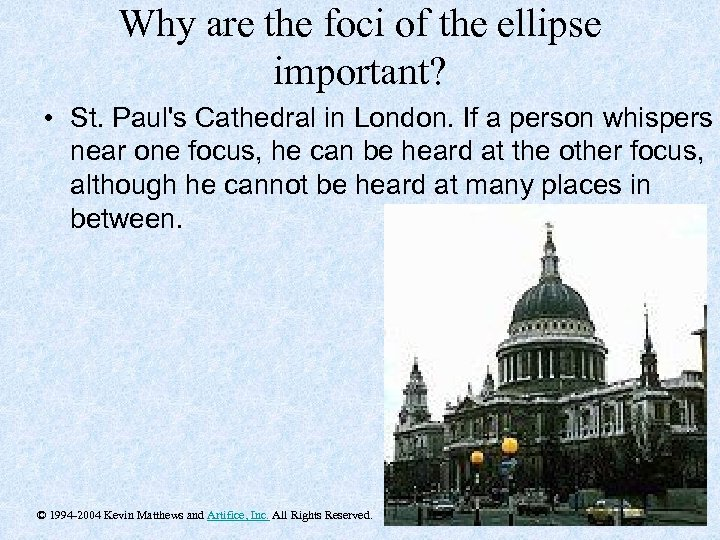Why are the foci of the ellipse important? • St. Paul's Cathedral in London. If a person whispers near one focus, he can be heard at the other focus, although he cannot be heard at many places in between. © 1994 -2004 Kevin Matthews and Artifice, Inc. All Rights Reserved.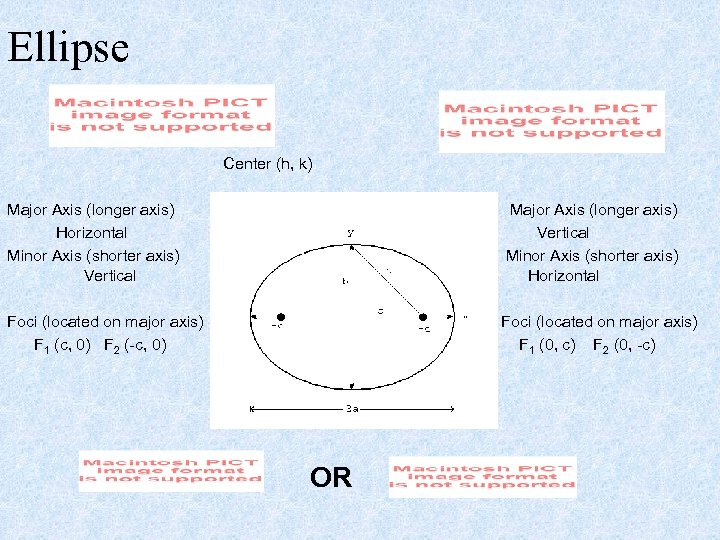Ellipse Center (h, k) Major Axis (longer axis) Horizontal Minor Axis (shorter axis) Vertical Major Axis (longer axis) Vertical Minor Axis (shorter axis) Horizontal Foci (located on major axis) F 1 (c, 0) F 2 (-c, 0) Foci (located on major axis) F 1 (0, c) F 2 (0, -c) OR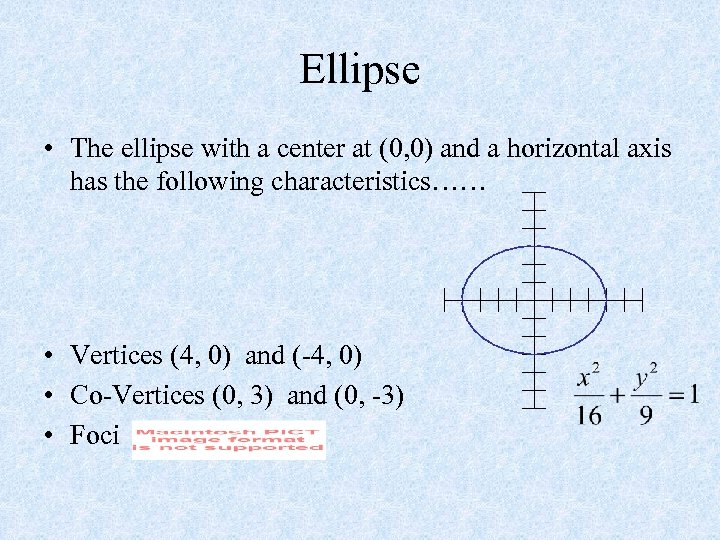Ellipse • The ellipse with a center at (0, 0) and a horizontal axis has the following characteristics…… • Vertices (4, 0) and (-4, 0) • Co-Vertices (0, 3) and (0, -3) • Foci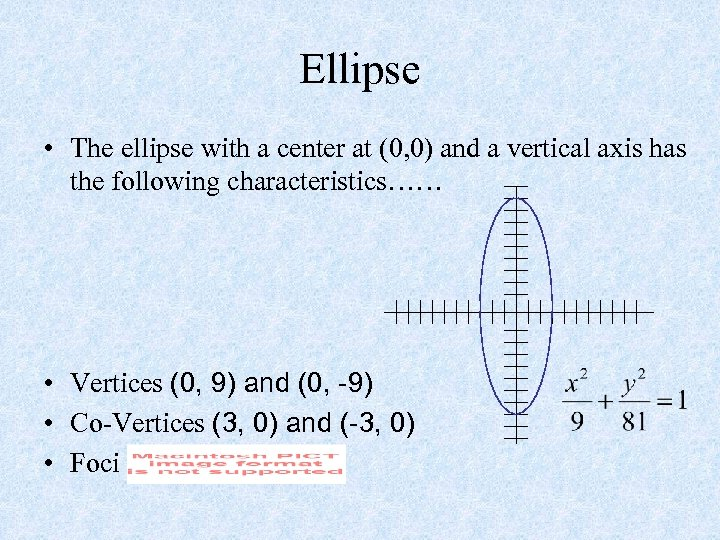Ellipse • The ellipse with a center at (0, 0) and a vertical axis has the following characteristics…… • Vertices (0, 9) and (0, -9) • Co-Vertices (3, 0) and (-3, 0) • Foci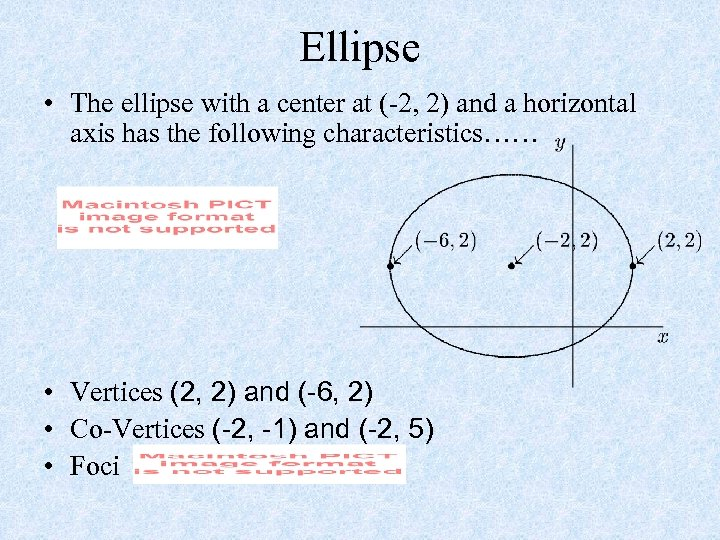Ellipse • The ellipse with a center at (-2, 2) and a horizontal axis has the following characteristics…… • Vertices (2, 2) and (-6, 2) • Co-Vertices (-2, -1) and (-2, 5) • Foci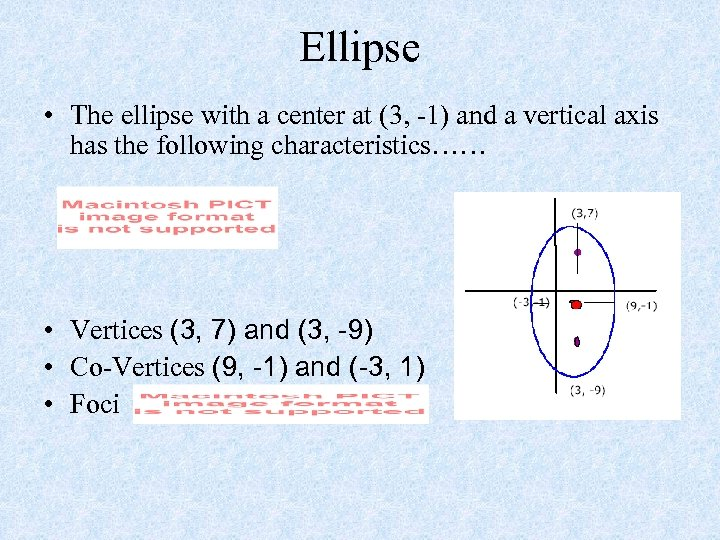Ellipse • The ellipse with a center at (3, -1) and a vertical axis has the following characteristics…… • Vertices (3, 7) and (3, -9) • Co-Vertices (9, -1) and (-3, 1) • Foci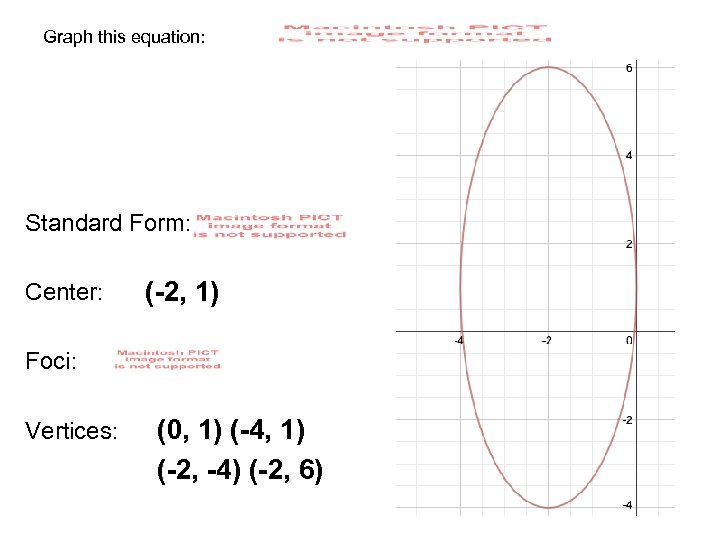Graph this equation: Standard Form: Center: (-2, 1) Foci: Vertices: (0, 1) (-4, 1) (-2, -4) (-2, 6)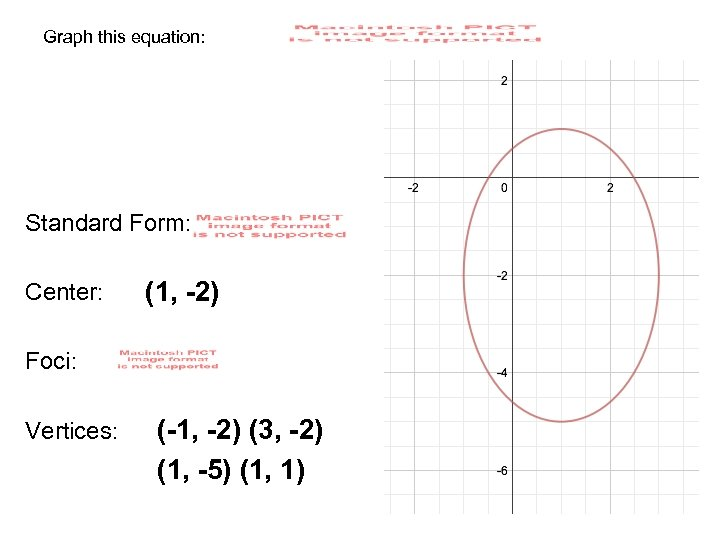Graph this equation: Standard Form: Center: (1, -2) Foci: Vertices: (-1, -2) (3, -2) (1, -5) (1, 1)Problems Graph 4 x 2 + 9 y 2 = 4 Find the vertices and foci of an ellipse: sketch the graph 4 x 2 + 9 y 2 – 8 x + 36 y + 4 = 0 put in standard form find center, vertices, and foci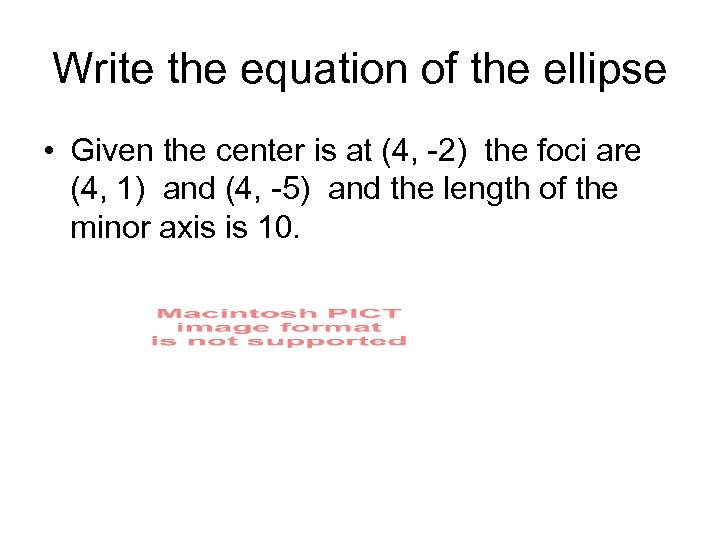Write the equation of the ellipse • Given the center is at (4, -2) the foci are (4, 1) and (4, -5) and the length of the minor axis is 10.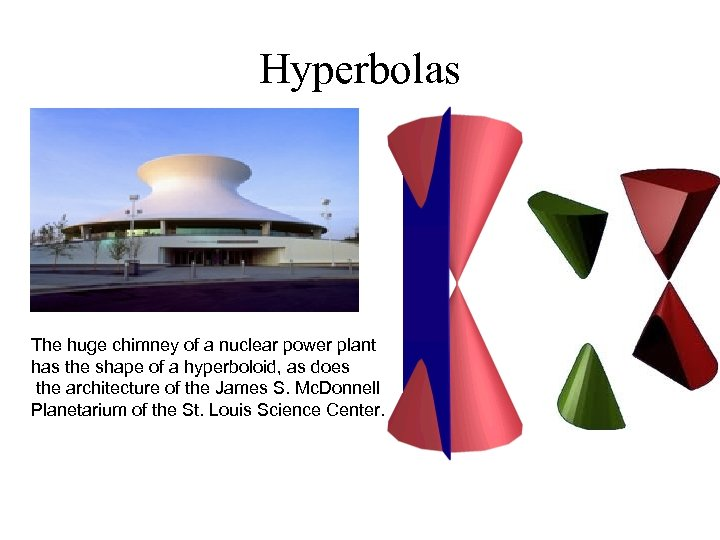Hyperbolas The huge chimney of a nuclear power plant has the shape of a hyperboloid, as does the architecture of the James S. Mc. Donnell Planetarium of the St. Louis Science Center.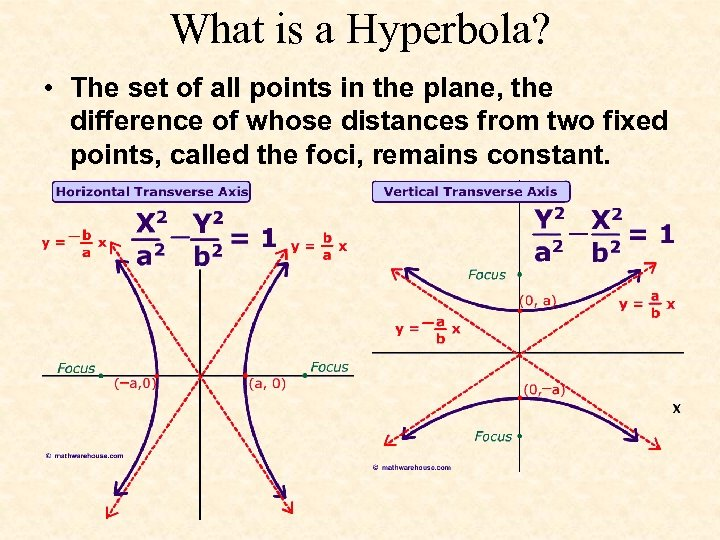What is a Hyperbola? • The set of all points in the plane, the difference of whose distances from two fixed points, called the foci, remains constant.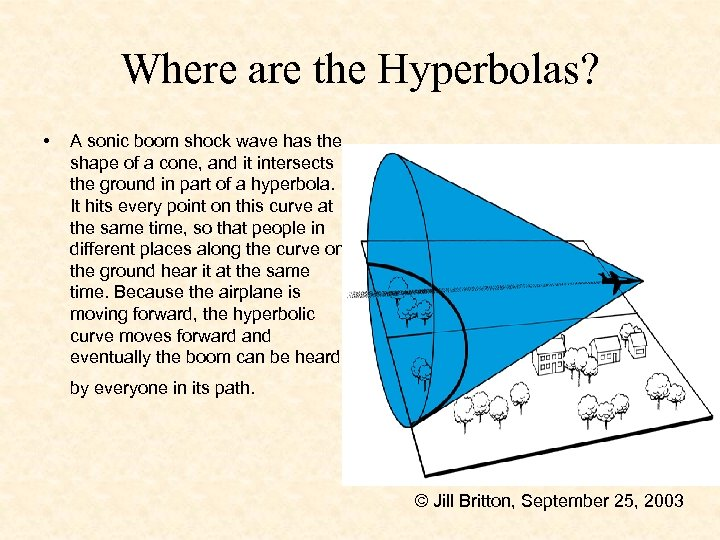Where are the Hyperbolas? • A sonic boom shock wave has the shape of a cone, and it intersects the ground in part of a hyperbola. It hits every point on this curve at the same time, so that people in different places along the curve on the ground hear it at the same time. Because the airplane is moving forward, the hyperbolic curve moves forward and eventually the boom can be heard by everyone in its path. © Jill Britton, September 25, 2003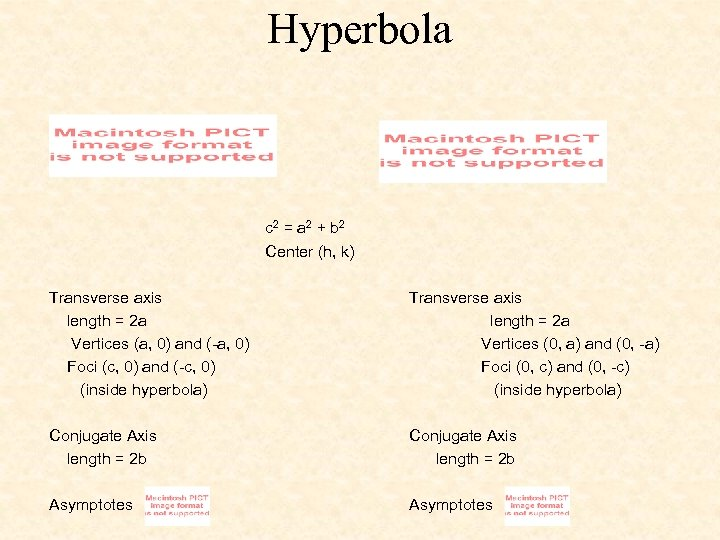Hyperbola c 2 = a 2 + b 2 Center (h, k) Transverse axis length = 2 a Vertices (a, 0) and (-a, 0) Foci (c, 0) and (-c, 0) (inside hyperbola) Transverse axis length = 2 a Vertices (0, a) and (0, -a) Foci (0, c) and (0, -c) (inside hyperbola) Conjugate Axis length = 2 b Asymptotes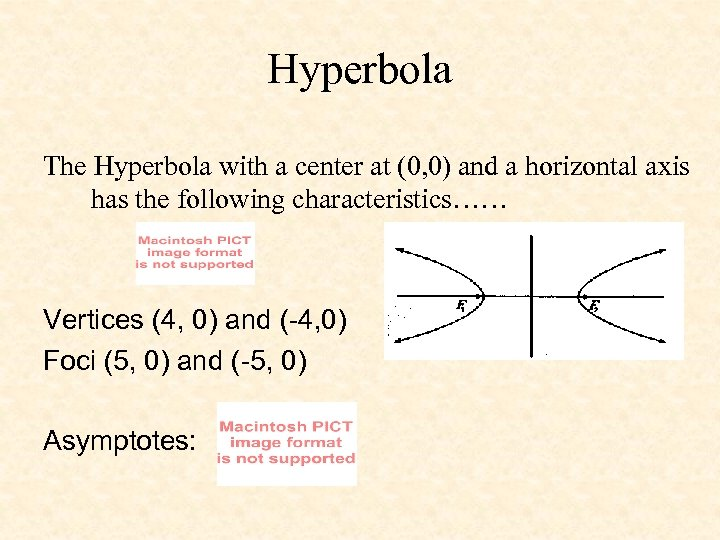Hyperbola The Hyperbola with a center at (0, 0) and a horizontal axis has the following characteristics…… Vertices (4, 0) and (-4, 0) Foci (5, 0) and (-5, 0) Asymptotes: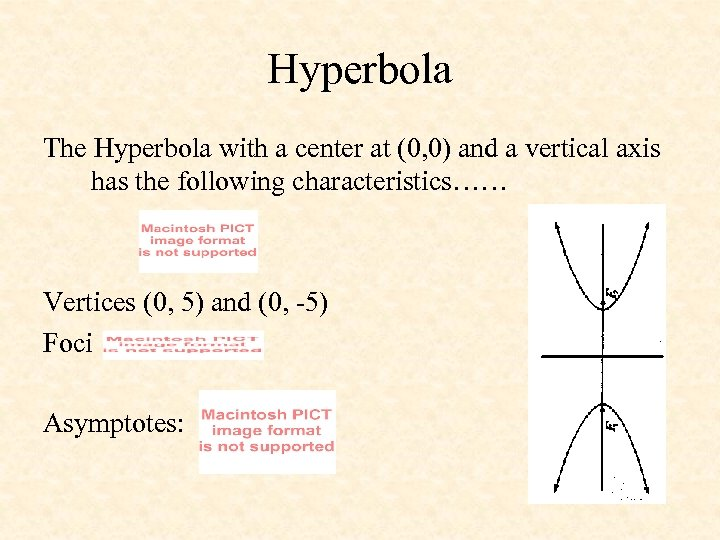Hyperbola The Hyperbola with a center at (0, 0) and a vertical axis has the following characteristics…… Vertices (0, 5) and (0, -5) Foci Asymptotes: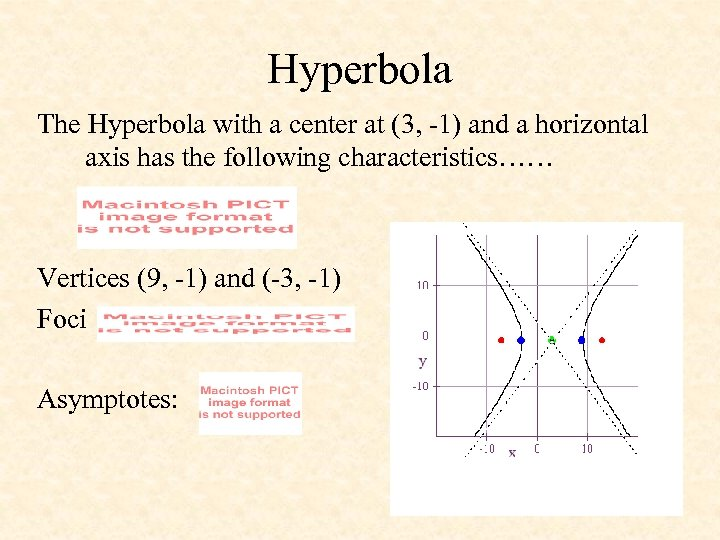Hyperbola The Hyperbola with a center at (3, -1) and a horizontal axis has the following characteristics…… Vertices (9, -1) and (-3, -1) Foci Asymptotes: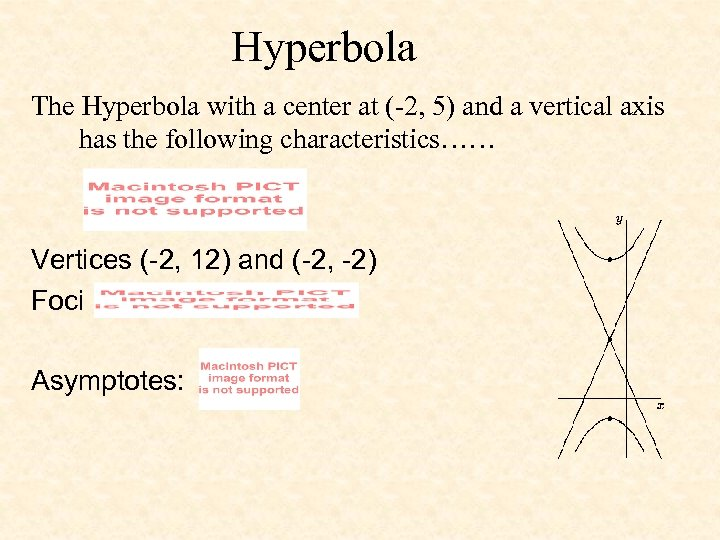Hyperbola The Hyperbola with a center at (-2, 5) and a vertical axis has the following characteristics…… Vertices (-2, 12) and (-2, -2) Foci Asymptotes: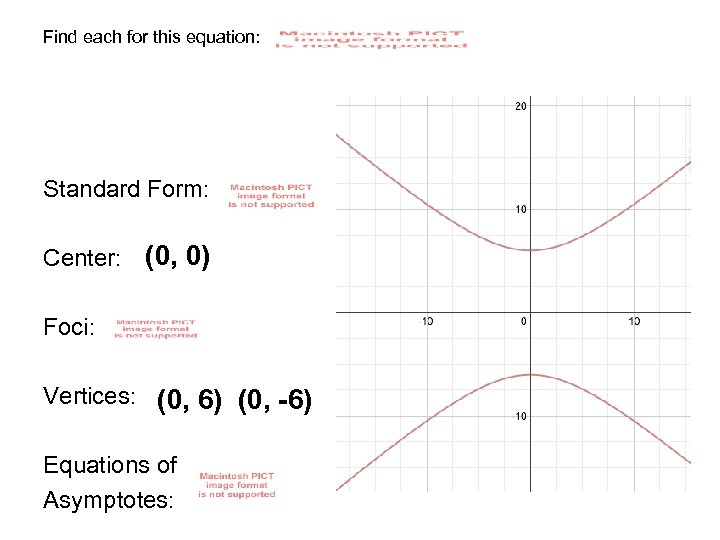Find each for this equation: Standard Form: Center: (0, 0) Foci: Vertices: (0, 6) (0, -6) Equations of Asymptotes: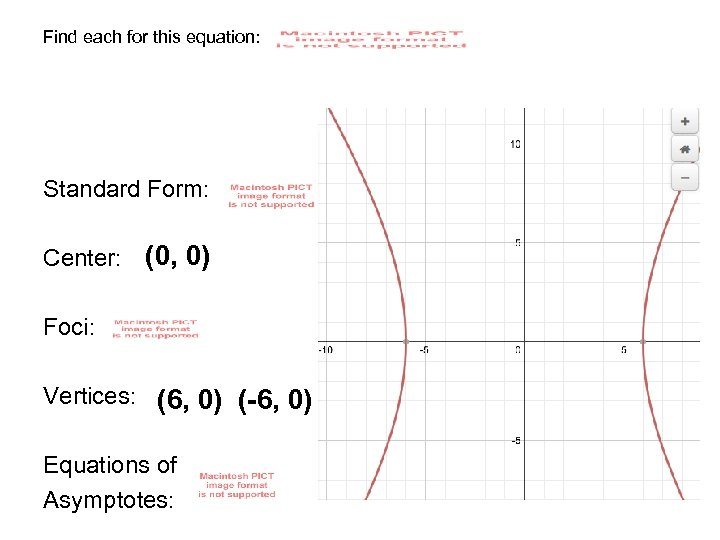Find each for this equation: Standard Form: Center: (0, 0) Foci: Vertices: (6, 0) (-6, 0) Equations of Asymptotes: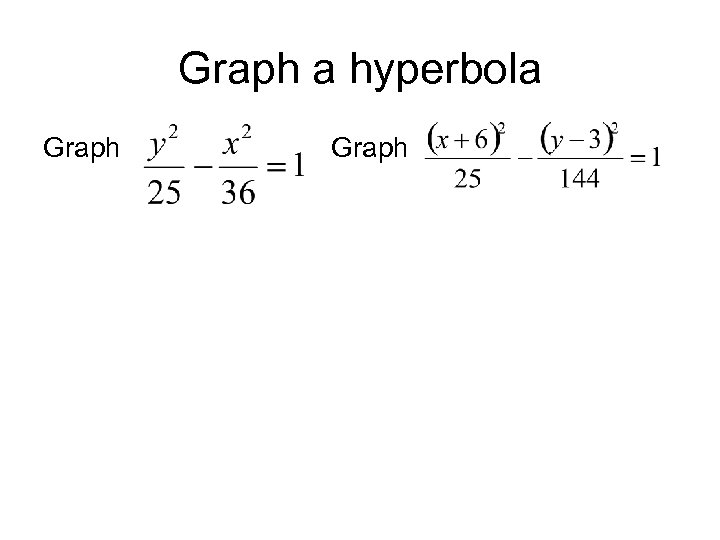Graph a hyperbola Graph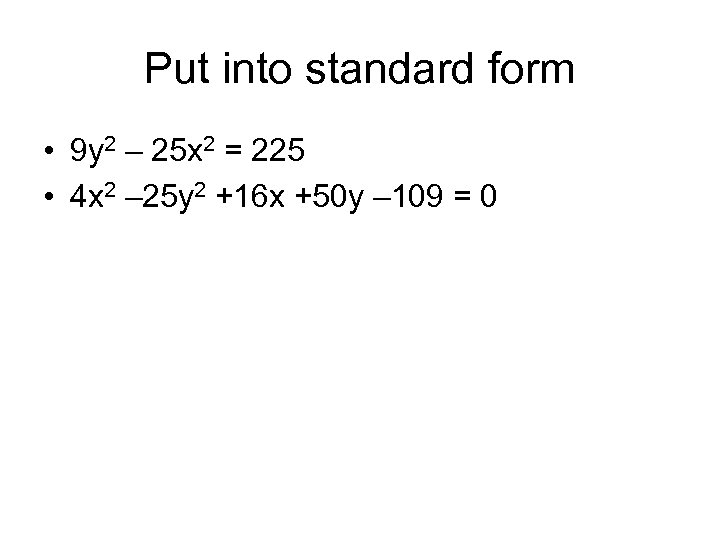Put into standard form • 9 y 2 – 25 x 2 = 225 • 4 x 2 – 25 y 2 +16 x +50 y – 109 = 0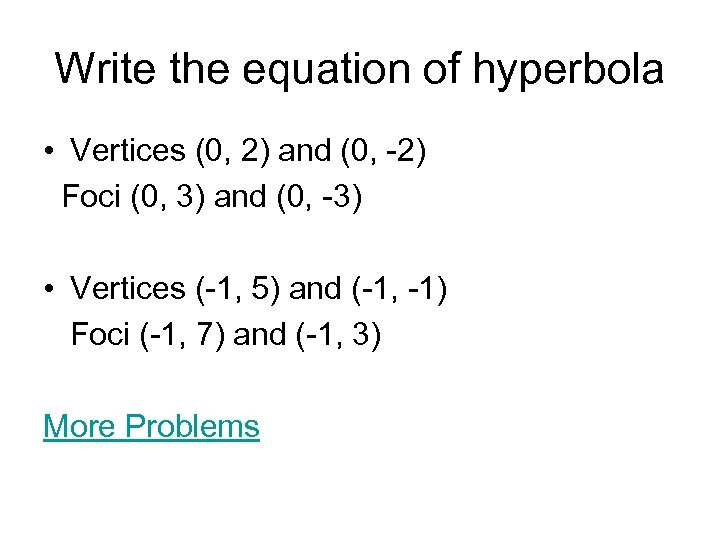Write the equation of hyperbola • Vertices (0, 2) and (0, -2) Foci (0, 3) and (0, -3) • Vertices (-1, 5) and (-1, -1) Foci (-1, 7) and (-1, 3) More Problems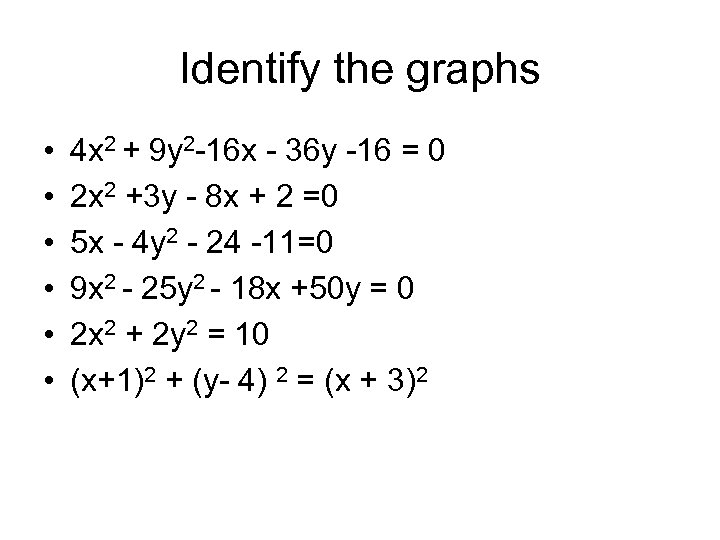Identify the graphs • • • 4 x 2 + 9 y 2 -16 x - 36 y -16 = 0 2 x 2 +3 y - 8 x + 2 =0 5 x - 4 y 2 - 24 -11=0 9 x 2 - 25 y 2 - 18 x +50 y = 0 2 x 2 + 2 y 2 = 10 (x+1)2 + (y- 4) 2 = (x + 3)2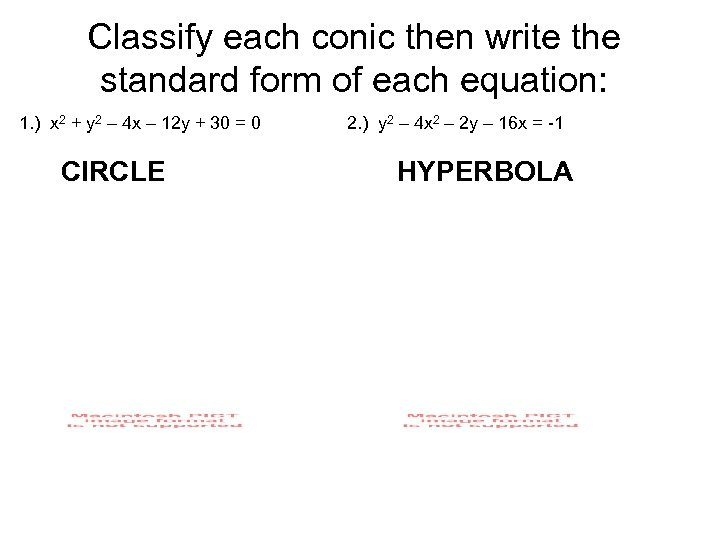Classify each conic then write the standard form of each equation: 1. ) x 2 + y 2 – 4 x – 12 y + 30 = 0 CIRCLE 2. ) y 2 – 4 x 2 – 2 y – 16 x = -1 HYPERBOLA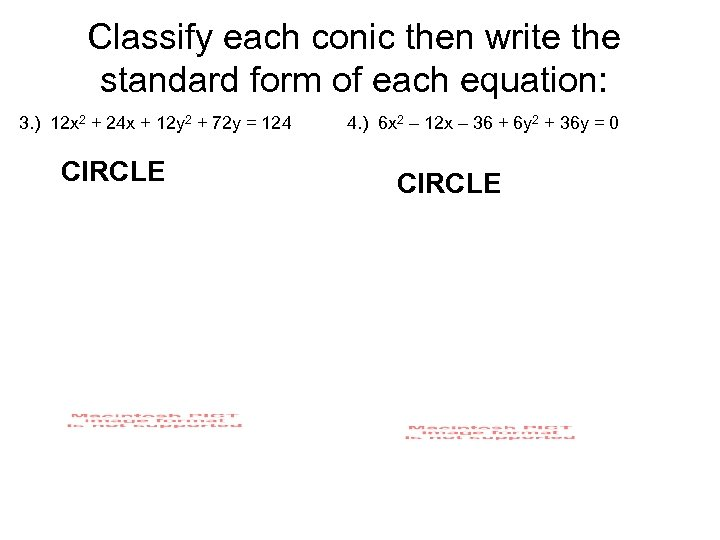Classify each conic then write the standard form of each equation: 3. ) 12 x 2 + 24 x + 12 y 2 + 72 y = 124 CIRCLE 4. ) 6 x 2 – 12 x – 36 + 6 y 2 + 36 y = 0 CIRCLE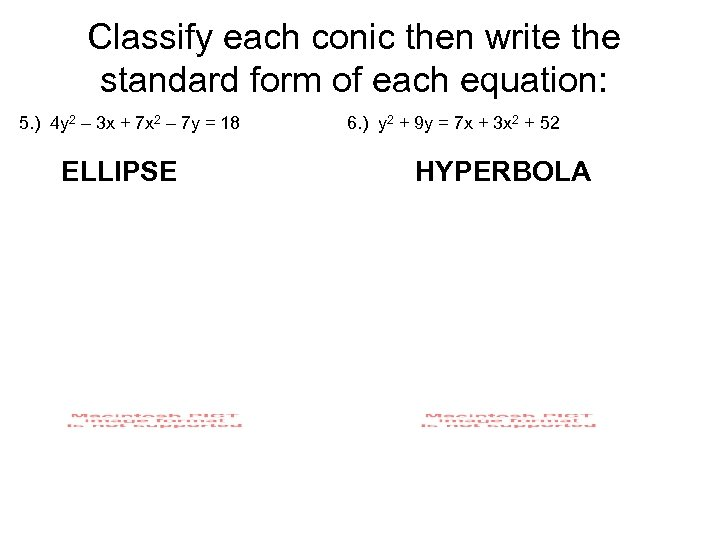Classify each conic then write the standard form of each equation: 5. ) 4 y 2 – 3 x + 7 x 2 – 7 y = 18 ELLIPSE 6. ) y 2 + 9 y = 7 x + 3 x 2 + 52 HYPERBOLA